Next: Replication Period Up: Detailed Analysis of a Previous: Detailed Analysis of a

## Program Length

The change in program length over the run is shown in Figure 5.2. A number of interesting points are apparent from this graph. The most obvious one is that there is a trend for increasing program length over the course of the run. The length of the ancestor programs was 348 bits, but by the end of the run, virtually all of the programs in the population were of length 390 bits.5.7 Recalling from the previous chapter that each instruction is represented by 6 bits, this increase in length corresponds to an extra 7 instructions being added to the ancestor.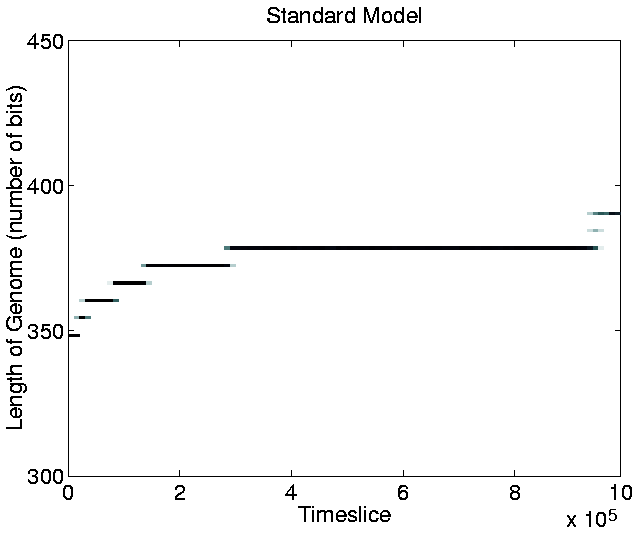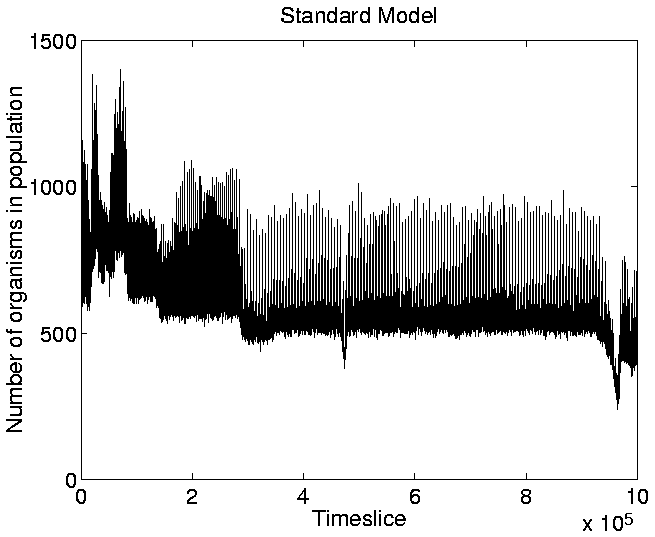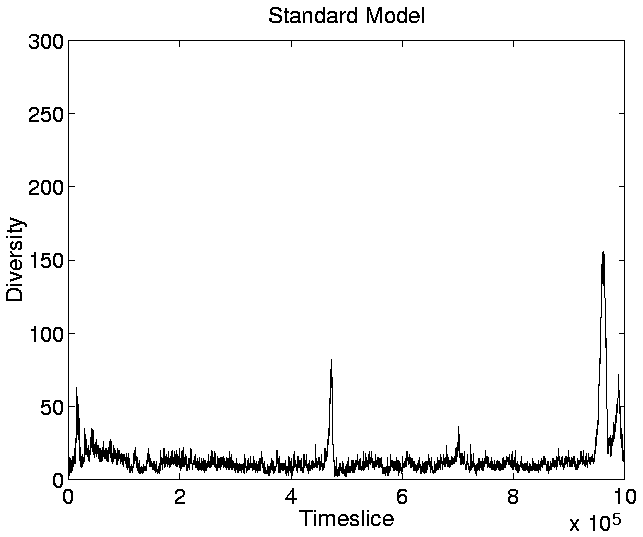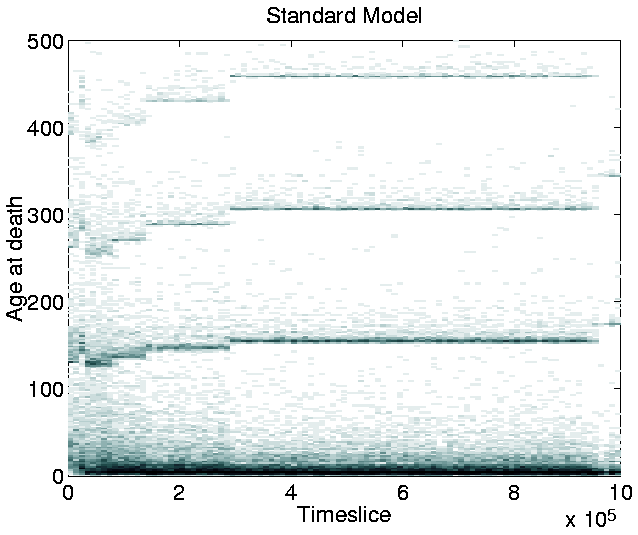Another point of interest in the graph is that, at any given time during the run, the population tends to be composed of organisms of only a single length.5.8 The only exception to this occurs during a transition phase from programs of one length to programs of a greater length. During these phases, progams of two, or, occasionally, three different lengths coexist, but the shorter programs soon die out, to be completely replaced by the longer ones.

This result is in contrast to those of similar runs on Tierra, where shorter parasite'' programs evolved and coexisted with the longer programs (as described in Section 3.2.1). As discussed in Section 3.3.1, the non-appearance of parasites was expected, because Cosmos does not allow cells to execute the code of other cells.

The increase in program length, on the other hand, was unexpected. During each timeslice, CPU-time was allocated to each program (if it had enough energy) according to its length, so that, all else being equal, selection was size neutral (see Section 4.2.3). However, the picture is complicated by the fact that the program has to cycle once round the copy loop'' to copy each instruction. In the ancestor, the copy loop accounts for 19 of the 58 instructions, or approximately one third of the total number. The total number of instructions, I, that must be executed to replicate the program is therefore approximately:

 (5.1)

where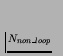is the number of instructions not involved in the copy loop (58-19=39), Nloop is the number of instructions in the copy loop (=19), and Ntotal is the total number of instructions (=58). In this case, I is therefore 39+19*58=1141 instructions. (This is not exactly right, because there are some conditional instructions, and the templates at the beginning and end of the program do not actually get executed, but it is near enough.) Now, during this run, the parameter et_value_constant was set to 0.025, so at each timeslice the ancestor (of length 58 instructions, or 58*6=348 bits) was allowed to run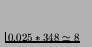instructions (rounded down to a whole number). The total number of timeslices required for an ancestor program to replicate itself is therefore approximately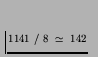timeslices.

Similar calculations can be made for programs of different lengths. If we assume that the size of the copy loop is always one third of the size of the whole program, the results we get are as shown in the left-hand side of Figure 5.8. In this case, longer programs still take longer to replicate, so we would still expect selection for shorter programs. (The graph looks somewhat erratic because of the effects of rounding down the number of instructions per time slice to an integer value, etc.) If however we assume that the size of the copy loop is constant no matter how long the program, the results are somewhat different (see the right-hand side of Figure 5.8). In this case, the assumption of length-neutral selection is more realistic.

As an increase in program length was observed, we might therefore expect the extra instructions to have been added outside of the copy loop. However, as the allocation of CPU-time is also dependent on a program having enough stored energy, it is possible that the observed increase in program length might be associated with the evolution of programs that collect and store more energy from the environment. In this case, it might even benefit programs to add more energy collection instructions inside the copy loop, as each one will then be executed during every iteration of the loop. The true cause of the increase in program length will be investigated in Section 5.2.7.Next: Replication Period Up: Detailed Analysis of a Previous: Detailed Analysis of a
Tim Taylor
1999-05-29